## The Binomial Theorem

by Kirby Urner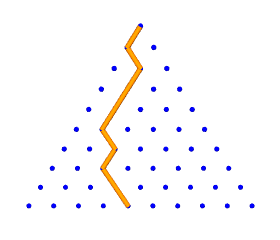Suppose I have two quantities a and b which vary independently of one another, meaning I cannot express one in terms of the other i.e. no operator accepting b as the only input will return a.

I need to keep a and b separately expressed, but I can still add and multiply them. And when either multiplies itself, I can use exponential notation, i.e. a * a = a^2.

Consider the quantity (a + b)^n -- I want to multiply (a + b) by itself n times, leaving a and b as algebraic variables. By the distributive property we know that:

```(a + b)(a + b) = a * (a + b) + b * (a + b)
= aa + ab + ba + bb
= a^2 + 2ab + b^2
```
Note that I can add terms with the same powerings of both a and b, i.e. ab and ba may be added, as can (a^3)b and b(a^3) (dropping the * for convenience).

Now what happens when I multiply the above result by (a + b) again? This will be a third powering of (a + b):

```(a + b)^3 = (a + b)(a^2 + 2ab + b^2)
= a * (a^2 + 2ab + b^2) +
b * (a^2 + 2ab + b^2)
```
All the terms in the second set of parentheses are going to be "promoted", first by a, then by b. By "promoted", I mean "multiplied by". So a^2 will be promoted to a^3, and 2ab to 2(a^2)b. I can write the results of promotion by a and b on two separate lines, lining up similar terms:
```a <--      a^3 + 2(a^2)b +  ab^2
(a^2)b + 2ab^2 + b^3    --> b
----------------------------
a^3 + 3(a^2)b + 3ab^2 + b^3
```
Notice how promotion by a and b results in the original terms shifting in the a and b directions, creating one new term at each end ("purely a" and "purely b"), while the middle terms line up and add.

I'll make this clearer by rewriting the above powerings using only coefficients i.e. how many of each kind of term:

```(a + b)                1  1  0
+  0  1  1
-------
(a + b)^2              1  2  1  0
+  0  1  2  1
----------
(a + b)^3              1  3  3  1  0
+  0  1  3  3  1
-------------
(a + b)^4              1  4  6  4  1
```
I can streamline the above yet further by writing each line of coefficents beneath the previous in a triangular format. This format is what many mathematicians call Pascal's Triangle, although it was known in China since at least the 1300s:
```Power
0                   1
1                 1   1
2               1   2   1
3             1   3   3   1
4           1   4   6   4   1
```
(a + b)^0 = 1 because any number raised to the 0 power is defined as 1.

Note that each row is obtained by adding the pair of numbers in the row above at the upper left and right whereas in the "wings" we only have a 1 in one corner, which propagates leftward or rightward.

So the next rows will read:

```Power
4          1    4    6    4    1
5        1    5   10   10    5    1
6      1    6   15   20   15   6     1
...
```
Here's a computer program which generates the coefficients up to a user-selected powering, by actually building Pascal's Triangle up to that row. Later, we will provide a short cut for doing this:
```* Program to generate Pascal's Triangle

* Note: some modifications will be required for
* powerings resulting in coefficients exceeding
* a width of 3 digits i.e. > 999.

oPascal = createobject("Pascal")
oPascal.generate(10)
oPascal.terms()
release objects
return

define class Pascal as custom
dimension nextrow(1), thisrow(1)
power  = 2
output  = ''

procedure generate(p)
with this
.power = p
* accept powering for final row and
* create two rows with power+1 terms each
dimension .nextrow(.power+1), .thisrow(.power+1)

* initialize both rows to all zeros
store 0 to .nextrow, .thisrow
* start at 1 and print it
store 1 to .thisrow(1)
?
?? str(1,3)
for n = 1 to .power
* the first term in the next row will be 1
.nextrow(1) = 1
?
?? str(.nextrow(1),3)
* all other next row terms are the sum of
* two successive terms in the previous row
for k = 2 to n+1
.nextrow(k) = .thisrow(k-1) + .thisrow(k)
?? "  " + str(.nextrow(k),3)
endfor
* make the next row be this row and loop
=acopy(.nextrow,.thisrow)
endfor
endwith
endproc

enddefine
```

Here's the output of the above program, taking us up to a 10th powering:

```  1
1    1
1    2    1
1    3    3    1
1    4    6    4    1
1    5   10   10    5    1
1    6   15   20   15    6    1
1    7   21   35   35   21    7    1
1    8   28   56   70   56   28    8    1
1    9   36   84  126  126   84   36    9    1
1   10   45  120  210  252  210  120   45   10    1
```
Returning the variables a and b to the picture, I will take the leftmost term as a raised to whatever power and decrease the a's power by 1 for every move to the right, and treat b symmetrically coming from the other direction:
```   (a + b)^6 =

1(a^6)(b^0)
+  6(a^5)(b^1)
+ 15(a^4)(b^2)
+ 20(a^3)(b^3)
+ 15(a^2)(b^4)
+  6(a^1)(b^5)
+  1(a^0)(b^6)
```
I've included 1 as a coefficient and 0 powers in the above total to make the pattern clearer. 1(a^0)(b^6) is more simply written as b^6.

If I had a function P(n,k) that returned the coefficients in Pascal's triangle, given simply the power n and item number k as input, then I could write something like:

```    (a + b)^n =

P(n,  1)(a^n  )(b^0  )
P(n,  2)(a^n-1)(b^1  )
P(n,  3)(a^n-2)(b^2  )
...
P(n,n-1)(a^2  )(b^n-2)
P(n,n  )(a^1  )(b^n-1)
P(n,n+1)(a^0  )(b^n  )
```
Even more compactly, I could use sigma notation, which is a way of looping through some code while incrementing a counter, and keeping a running total. In computer language, we would call this a kind of "do-loop":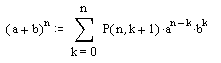Written as a do-loop:
```    for k = 0 to n
terms = terms + P(n,k+1) * (a^(n-k)) * (b^k)
endfor
```
Actually the computerized algorithm would have to be more explicit as what I'm doing here is expanding out (a+b)^n symbolically, leaving a and b as variables while evaluating the function P(n,k+1) to get a whole number coefficient. The computer would need to be instructed about these details.

Below is such a method. It depends on results from the above generate( ) method already stored in the array named thisrow. P(k) function simply looks up the coefficient previously stored in the array.

```procedure terms
with this
local k, astring, bstring, coeff
.output = ''
for k = 0 to .power
astring = "(a^"+str(.power-k,2)+")"
bstring = "(b^"+str(k,2)+")"
coeff   = str(.P(k+1),3)
? " + " + coeff + astring + bstring
.output = .output + " + " + coeff + astring + bstring
endfor
endwith
endproc

function P(k)
return this.thisrow(k)
endfunc
```
Here's what it prints out for n=10 i.e. (a+b)^10.
``` +   1(a^10)(b^ 0)
+  10(a^ 9)(b^ 1)
+  45(a^ 8)(b^ 2)
+ 120(a^ 7)(b^ 3)
+ 210(a^ 6)(b^ 4)
+ 252(a^ 5)(b^ 5)
+ 210(a^ 4)(b^ 6)
+ 120(a^ 3)(b^ 7)
+  45(a^ 2)(b^ 8)
+  10(a^ 1)(b^ 9)
+   1(a^ 0)(b^10)
```
What I'd really like is a this function P that doesn't force me to compute the whole triangle up to row n. Surely there must be a simpler rule that will generate each row "from scratch" without relying on the one above it.
```                   1
1   1
1   2   1
1   3   3   1
1   4   6   4   1
```
Looking at Pascal's Triangle again, I see what many mathematics and science texts have pointed out: if I think of each number as a "decision point" where a falling object has to fall leftward or rightward to the next row, then each number represents the number of pathways by which an object might have come to it.

For example, below we see the 3 ways a falling ball could reach the final destination, where the number 3 appears.

```       1                 1                 1
/                 /                   \
1   1             1   1             1   1
\               /                     /
1   2   1         1   2   1         1   2   1
/               \                   /
1   3   3   1     1   3   3   1     1   3   3   1
```

The endmost positions have a 1 in them because there is only one way to reach these extremes: a ball has to always fall left, all the way to the bottom, or always fall right. If we assume the probability of falling either left or right is equally likely, then of course balls will make it to these extreme positions relatively infrequently, and to the more centered positions with the greatest frequency.

As you might expect, this is where many math texts pause to make some remarks about the Bell Curve, graphed as a smoothly peaking hill which symmetrically tapers off towards extremely improbable outcomes on either side of a most probable peak. Here's a picture of said curve: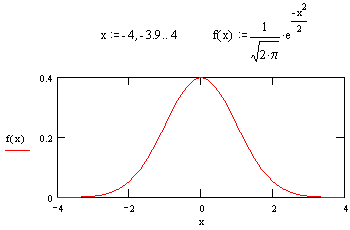Returning to our left/right falling ball, instead of saying "left" and "right" I might say it falls in either the a or b direction. This makes every route to a terminus describable in terms of the sequence of decisions to fall a-ward or b-ward.

Each route to the same position contains the same total numbers of a's and b's, but their order changes.

```       1                 1                 1
/                 /                   \
1   1             1   1             1   1
\               /                     /
1   2   1         1   2   1         1   2   1
/               \                   /
1   3   3   1     1   3   3   1     1   3   3   1

a                 a                 b
b                 a                 a
a                 b                 a
```
The coefficient at the terminus represents all possible orders of a's and b's, the number of each depending on the terminus. The exponents of a and b, (n-k) and k, give us the numbers of a's and b's actually associated with a coefficient. For example, the 3 shown above is the coefficient of aab, i.e. (a^2)b.

So, given aaaabb (four a's and 2 b's) how many distinct arrangements are possible? If I can figure out a general rule for the number of distinct arrangements of n things where k of them are b and n-k are a (k is between 0 and n), then I'll have the short-hand P( ) function for which I'm looking.

The first a might be in any of n positions, leaving n-1 vacancies. The next a might go in any one of those, until I have just one a left and k+1 remaining positions from which to choose. I can write this as n * (n-1) * ... * (k+1) or n!/k!. My b's will appear in all the remaining k positions not occupied by an a.

```n     = 6 (total number of things)
k     = 2 (number of b's)
(n-k) = 4 (number of a's)

. . . . . .  <-- 6 positions for the 1st a    6

. a . . . .  <-- 5 positions for the next a   6 x 5

. a . . a .  <-- 4 remaining                  6 x 5 x 4

a a . . a .  <-- 3 remaining (= k + 1)        6 x 5 x 4 x 3 = 360

a a . a a .  <-- all n-k used

a a b a a b  <-- fill in the rest with b's
```
But the above sequence, aabaab, would have been reached if I had put my a's in these same positions starting with my first a in position 5, the next in position 1... lots of ways to end up with this same sequence. In other words, I've combined four unique rows, each containing an a, but I can order these rows in 4 x 3 x 2 x 1 ways, i.e. in (n-k)! ways.
```. a . . . .    any of 4 rows first, then any of 3, any of
. . . . a .    2 = 4 x 3 x 2 combinations leading to the
a . . . . .    same result (a a b a a b)
. . . a . .
-----------
a a b a a b
```
So I need to divide 360 by 24 in the above example, getting 15. In general, I need to obtain n!/k! and then divide further by (n-k)!.
```Power
5        1    5   10   10    5    1
6      1    6   15   20   15    6    1
k = 0    1    2    3    4    5    6
^
15(a^4)b^2  <-- term
```
So the complete expression we're looking for is n!/k!(n-k)! where k ranges from 0 to n.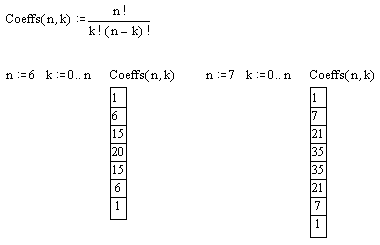I can use this new expression to subclass my previously defined Pascal class, and swap in new code for P( ). This will allow me to output an expansion of (a+b)^n without first generating all the rows of Pascal's Triangle up to the row for the nth power.

Since the computer language I'm using doesn't have a built in function for factorial, I have to write code for that too. Note also that my previous terms( ) function passes k+1 to the P( ) method, because it was written to point to a previously stored array (this language indexes arrays starting at 1).

Since I don't want to rewrite terms( ) for this subclass -- I'd rather just inherit the existing method from the superclass -- I take care of the problem by decrementing the value passed to my new P( ) method, writing term = term - 1.

```oPascal = createobject("fastPascal")
oPascal.power = 7
oPascal.terms()
release objects
return

define class fastPascal as Pascal

function P(term)
term = term - 1
with this
return .fact(.power)/(.fact(.power - term) * .fact(term))
endwith
endfunc

function fact(n)
local i, output
output = 1
for i = 2 to n
output = output * i
endfor
return output
endfunc

enddefine
```
Here's the output generated by the above code (and the superclass upon which it depends):
``` +   1(a^ 7)(b^ 0)
+   7(a^ 6)(b^ 1)
+  21(a^ 5)(b^ 2)
+  35(a^ 4)(b^ 3)
+  35(a^ 3)(b^ 4)
+  21(a^ 2)(b^ 5)
+   7(a^ 1)(b^ 6)
+   1(a^ 0)(b^ 7)
```
These are indeed the coefficients I expected. We now can write a more generic expression for the binomial theorem, giving more explicit computations for P(n,k) in our sigma notation: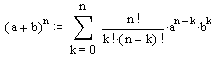Sir Isaac Newton is often given credit for first obtaining this result.

 An interesting segue to other math topics, often cited in the literature, is the fact that Pascal's Triangle also serves to generate successive terms of the Fibonacci series, as shown at right. The Fibonacci series results from adding the two previous terms to get the next, starting with 1, 1. The 3rd term is 1 + 1 = 2. The 4th term is 1 + 2 = 3, then 2 + 3 = 5, 3 + 5 = 8 and so on.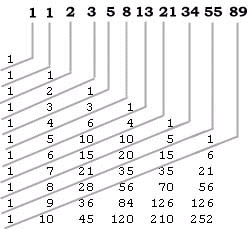The Fibonnaci series is in turn often used as a segue into investigations involving the Golden Mean or Phi. The value of Phi is algorithmically approached as the ratio Fib(N+1)/Fib(N), where Fib(N) and Fib(N+1) represent any two successive terms in the series. As N increases, the ratio converges to Phi, or (1 + Root(5))/2. ```Ratio Floating Point (15 decimals) ----- ---------------------------- 1/ 1 = 1.000000000000000 2/ 1 = 2.000000000000000 3/ 2 = 1.500000000000000 5/ 3 = 1.666666666666667 8/ 5 = 1.600000000000000 13/ 8 = 1.625000000000000 21/13 = 1.615384615384615 34/21 = 1.619047619047619 55/34 = 1.617647058823529 89/55 = 1.618181818181818 ... Phi = 1.618033988749895 ``` For further reading: Return to Main Outline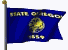Home Page